×#### Thank you for registering.

One of our academic counsellors will contact you within 1 working day.

Click to Chat

1800-1023-196

+91-120-4616500

CART 0

• 0

MY CART (5)

Use Coupon: CART20 and get 20% off on all online Study Material

ITEM
DETAILS
MRP
DISCOUNT
FINAL PRICE
Total Price: Rs.

There are no items in this cart.
Continue Shopping• Complete JEE Main/Advanced Course and Test Series
• OFFERED PRICE: Rs. 15,900
• View Details

```Revision Notes on Circles

CircleA figure made by all the points which are at the same distance from a fixed point is called a Circle.

1. Center

The fixed point is known as the centre of the circle.

The distance from any point on the circle to the fixed point is the radius. Any line segment which joins the centre and any point on the circle is known as the Radius.

3. Chord

Any line segment made by joining any two points on the boundary of the circle is called Chord.

4. Diameter

Diameter is two times the radius. It is the longest chord on the circle which passes through the centre. All the diameters have the same length.

5. Circumference

The length of the boundary of the circle is called the circumference of the circle.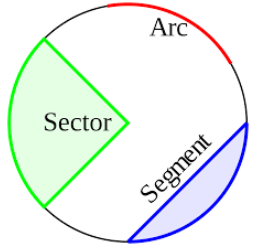6. Arc

An arc is the part of the circle joining two points on the circumference of the circle.

7. Sector

An area made by an arc and two radii of the circle, by joining the centre to the endpoints of the arc is called Sector.

8. Segment

An area made by a chord and an arc of the circle is called Segment.

The Relation between a Circle and a Line in a Plane

There could be three situations when there are a line and a circle.1. Non-intersecting Line

When a line and a circle have no common point then it is called a Non-intersecting Line with respect to the circle.

2. Secant

When a line intersects a circle in such a way that there are two common points then that line is called Secant.

3. Tangent

When a line touches the circle in such a way that they have only one common point then that line is called a Tangent. That common point is called the point of contact.

Tangent to a Circle

All the tangents of a circle are perpendicular to the radius through the point of contact of that tangent.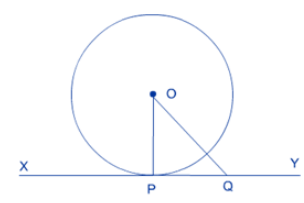OP is the radius of the circle and Q is any point on the line XY which is the tangent to the circle. As OP is the shortest line of all the distances of the point O to the points on XY. So OP is perpendicular to XY. Hence, OP⊥ XY

Example

Find the radius of the circle in the given figure, if the length of the tangent from point A which is 5 cm away from center is 4 cm.

Solution

As we know that the radius is perpendicular to the radius, so the ∆ABO is a right angle triangle.

Given, AO = 5 cm and AB = 4 cm

We can use Pythagoras theorem here

OA2 = OB2 + AB2

OB2 = OA2 - AB2

= 52 - 42

= 25 – 16

OB2 = 9

OB = 3

So the radius of the given circle is 3 cm

Number of Tangents from a Point on a Circle

1. There could be only one tangent at one point of contact.

2. Tangent could not be drawn from any point inside the circle.

3. There could be only two tangents to a circle from any point outside the circle.

The lengths of tangents drawn from an external point to a circle are equal.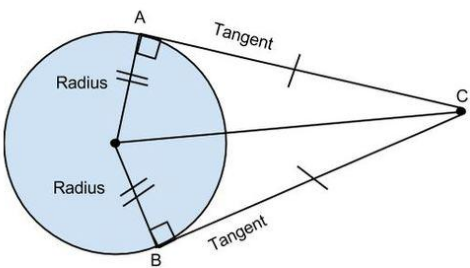Here, two tangents are drawn from the external point C. As the tangent is perpendicular to the radius, it formed the right angle triangle.

So ∆AOC and ∆BOC are congruent right angle triangle. Hence AC = BC.

Example

If two tangents PA and PB are drawn to a circle from a point P with centre O and OP is equal to the diameter of the circle then show that triangle APB is an equilateral triangle.Solution

Given, AP is a tangent to the circle.

Therefore, OA ⊥ AP (Tangent is perpendicular to the radius through the point of contact)

∠OAP = 90°

In ∆OAP,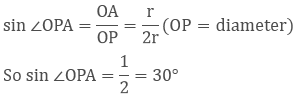Likewise, we can prove that ∠OPB = 30°

Now, ∠APB = ∠OPA + ∠OPB = 30° + 30° = 60°

In ∆PAB, PA = PB (length of the tangents from the external point is equal)

∠PAB = ∠PBA (Angles opposite to equal sides are equal)

∠PAB + ∠PBA + ∠APB = 180° (Due to angle sum property)

∠PAB + ∠PBA = 180° - 60°

2∠PAB = 120

∠PAB = 60°

As ∠PAB = ∠PBA = ∠APB = 60°

Hence, PAB is an equilateral triangle.

Example

Find the length of AB in the given circle, which is the chord in the outer circle and tangent to the inner circle. The radius of the inner and outer circle is 6 cm and 10 cm respectively.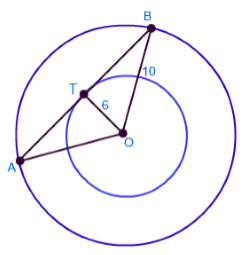Solution

Given

Radius of the inner circle (r) = 6 cm

Radius of outer circle (R) = 10 cm

As the Point T which is the tangent point is the midpoint of the chord, AT = TB

As radius is perpendicular to the tangent,

So is a right angle triangle and we can use Pythagoras theorem.

OB2 = OT2 + TB2

TB2 = OB2 - OT2

= 102 - 62

= 100 – 36

TB2 = 64

TB = 8 cm

AB = TB + AT

AB = 8 + 8 (AT = BT)

AB = 16 cm
```### Course Features

• 728 Video Lectures
• Revision Notes
• Previous Year Papers
• Mind Map
• Study Planner
• NCERT Solutions
• Discussion Forum
• Test paper with Video Solution